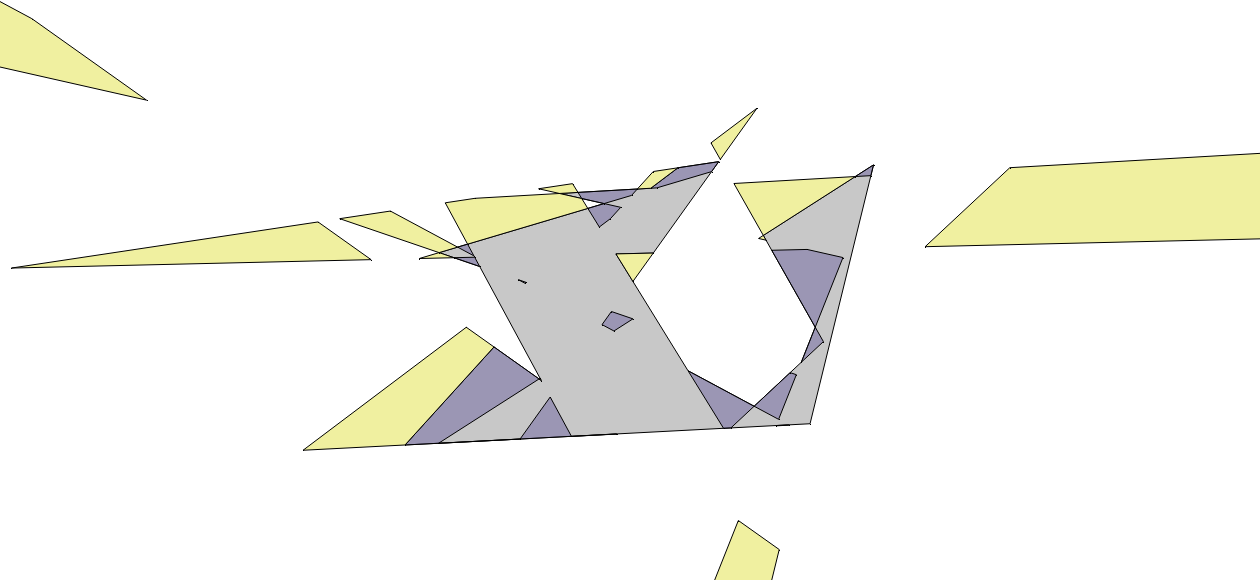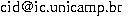IC-UNICAMP

# NWLPLIB - Wireless Localization Problem Library, by B. E. Crepaldi, P. J. de Rezende and C. C. de Souza

Use the BibTeX entry:

@Misc{wireless-localization-instances-page,
author = {Bruno E. Crepaldi and Pedro J. de Rezende and Cid C. de Souza},
title = {Instances for the {Natural Wireless Localization Problem}},
year = {2013},
note = {\sl{www.ic.unicamp.br/\$\sim\$cid/Problem-instances/Wireless-Localization}}
}

### Short problem description

The Natural Wireless Localization Problem (NWLP) is a variation of the art galley problem and is proved to be NP-hard. Given a simple polygon P, we are asked to determine the minimum number of broadcast antennas sufficient to determine for any point in the plane whether this point is inside or outside P. The antennas are limited to lie on vertices or edges of P and to transmit their signals within the range corresponding to the interior angle of the polygon at the point where they are positioned.

### What is in this library

NWLPLIB is a library of sample instances for the WLP problem consisting of random simple polygons of multiple sizes.

For all instances available below, the corresponding results are also shown.

### File Format: Instances

Each file consists of one line divided in two parts. The first part is an integer value that represents the number of vertices of the polygon.
The second part is a counterclockwise sequence of the vertices. Each vertex is represented by its x and y coordinates each of which is written as the quotient of two integers int/int.

As an example, here is the representation of a square with coordinates (1, 1) (50, 1) (50, 50) and (1, 50):
` 	4   1/1   1/1   100/2   1/1   500/10   50/1   1/1   100/2`

### File Format: Results

```	vertices: (integer with the number of vertices in the instance)
total_faces: (integer with the number of faces in the planar subdivision)
internal_faces: (integer with the number of internal faces in the planar subdivision)
internal_shadow_faces: (integer with the number of internal shadow faces in the planar subdivision)
external_faces: (integer with the number of external faces in the planar subdivision)
external_light_faces: (integer with the number of external light faces in the planar subdivision)
guards_used: (integer with the number of guards/antennas in the optimal solution)
iterations: (integer with the number of iterations used in the iterative algorithm)
complete_model_constraints: (integer with the number of constraints in the complete model)
first_iterations_constraints: (integer with the number of constraints in the first iteration of the algorithm)
last_iterations_constraints: (integer with the number of constraints in the last iteration of the algorithm)
time_solution_phase: (double with the time, in seconds, used by the solution phase)
time_preprocessing_phase: (double with the time, in seconds, used by the preprocessing phase)```

### Instances CCCG 2013

Instances and results presented in the paper An Efficient Exact Algorithm for the Natural Wireless Localization Problem, B. E. Crepaldi, C. C. de Souza and P. J. de Rezende, 2008, in Proceedings of CCCG2013 .

A total of 300 random simple polygons instances, each one having between 20 and 600 vertices:

These results correspond to runs executed on: AMD Phenom II X6 1055T @ 2.80GHz and 8GB RAM, CGAL 4.1, IBM ILOG CPLEX 12.2.

### Instances CGTA 2013

Instances and results presented in the paper Solving the Natural Wireless Localization Problem to Optimality Efficiently, B. E. Crepaldi, C. C. de Souza and P. J. de Rezende, 2008, submitted.

A total of 720 random simple polygons instances, including polygons with holes, each one having between 20 and 1000 vertices:

These results correspond to runs executed on: AMD Phenom II X6 1055T @ 2.80GHz and 8GB RAM, CGAL 4.1, IBM ILOG CPLEX 12.2.

### Example of an InstanceSimple Polygon with 20 vertices.Part of the induced arrangement.Some internal shadow and external light faces highlighted.

e-mail: